# Math in Focus Grade 8 Cumulative Review Chapters 7-9 Answer Key

This handy Math in Focus Grade 8 Workbook Answer Key Cumulative Review Chapters 7-9 detailed solutions for the textbook questions.

## Math in Focus Grade 8 Course 3 B Cumulative Review Chapters 7-9 Answer Key

Concepts and Skills

Find the value of each variable. (Lesson 7.1)

Question 1.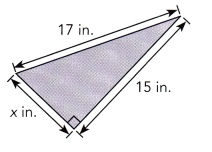Pythagorean Theorem states that the sum of the squares of the legs is the square of hypotenuse of a right angle. Let x be the unknown length in the right angle so,
x² + 15² = 17²
x² + 225 = 289
x² = 289 – 225
x² = 64
x = 8 (Square root of 64)
Therefore, the leg is 8 inches.

Question 2.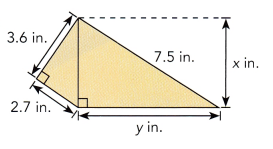(3.6)² + (2.7)² = x²
12.96 + 7.29 = x²
20.25 = x²
4.5 = x (Square root of 20.25)
Therefore, x is 4.5 inches.

The side lengths of a triangle are given. Decide whether each triangle is a right triangle. (Lesson 7.1)

Question 3.
6 cm, 8 cm, 12 cm
6² + 8² = 12²
36 + 64 = 144
100 = 144 (100 is not equal to 144)
Since the sum of the square of the legs is not equal to the square of hypotenuse, h=the triangle is not a right triangle.

Question 4.
9 in., 7.2 in., 5.4 in.
(7.2)² + (5.4)² = (9)²
51.84 + 29.16 = 81
81 = 81
Therefore, the triangle is a right triangle.

Find the distance between each pair of points. If necessary, round your answer to the nearest tenth of a unit. (Lesson 7.2)

Question 5.
P(2, 5), Q(4, 13)
d = √(x2 – x1)² + (y2 – y1)²
d = √(4 – 2)² + (13 – 5)²
d = √(2)² + (8)²
d = √4+64
d = √68
d = ±8.2

Question 6.
X(3, -1), Y(4, 2)
d = √(x2 – x1)² + (y2 – y1)²
d = √(4 – 2)² + (2 – (-1))²
d = √(2)² + (3)²
d = √4+9
d = √13

For each solid, find the unknown dimension. If necessary, round your answer to the nearest tenth of a unit. (Lesson 7.3)

Question 7.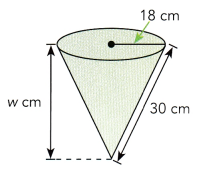Using pythagorean theorem
18² + w² = 30²
324 + w² = 900
w² = 900 – 324
w² = 536
w ~ 23.2 (Square root of 536)
Therefore, the height w of the cone measures 23.2 cm.

Question 8.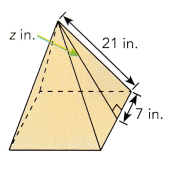Using pythagorean theorem,
z² + 7² = 21²
z² + 49 = 441
z² = 441 – 49
z² = 392
z = 19.8 (Square root of 392)
Therefore, the height is 19.8 inches.

Find the volume of each composite solid. Use 3.14 as an approximation for π. If necessary, round your answer to the nearest tenth. (Lesson 7.4)

Question 9.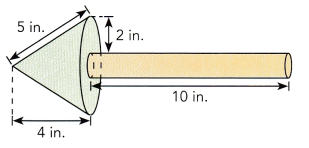Finding the radius of the cone:
4² + n² = 5²
16 + n² = 25
n² = 25 – 16
n² = 9
n = 3 ( square root of 9 )
Therefore, the radius of the cone is 3 inches. Deducting 2 inches to radius of the cone determines the radius of the cylinder according to the diagram. Then the radius of the cylinder is 1 inch.
Finding volume of the cone:
For the volume of the cone, using 3.14 as the value of π and let h be the height,
Volume of the cone = 1/3x Area of the base x Height of the cone
= 1/3 πr² x h
= 1/3 x 3.14 x (3)² x h
= 37.68
Therefore, the cone has a volume of 37.68 cubic meters.
Finding the volume of cylinder:
For the volume of the cylinder, using 3.14 as the value of π and the height j measures 10 inches.
Volume of the cylinder = Area of the base x height of cylinder
= πr² x j
= 3.14 x (1)² x 10
= 31.4
Therefore, the cylinder has a volume of 31.4 cubic inches.
By adding two volumes will get the total volume of the figure,
Total volume = Volume of the cone + Volume of the cylinder
= 37.68 + 31.4
= 69.08
Therefore, the total volume is 69.08 cubic meters.

Question 10.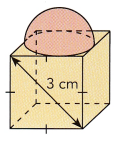Finding the sides of the cube:
g² + h² = 3²
g² + h² = 9
g² + g² = 9 (since g = h)
2 g² = 9
g² = 9/2
g² = 4.5
g ~ 2.1(Square root of 4.5)
Therefore, the sides of the cube measures 2.1 cm.
Finding the volume of the cube:
Volume of the cube = s³
= (2.1)³
~ 9.3
Therefore, the volume of the cube is 9.3 cubic cm.
Finding the volume of the sphere:
Volume of the sphere = 4/3 πr³
= 4/3 x 3.14 x (1.05)³
= 4/3 x 3.14 x 1.2
= 4.8
Volume of the hemisphere = Volume of the sphere/2
= 4.8/2
= 2.4
Therefore, the hemisphere has a volume of 2.4 cubic cm.

Find the coordinates of the image under each translation. (Lesson 8.1)

Question 11.
A (3, -2) is translated 1 unit to the right and 8 units up.
Answer: Locate the point (3, -2) in the coordinate plane then move it 1 unit to the right and 8 times upwards as described by the translation. Thus the image for A will be on points (4,6) on the coordinate plane. Therefore, A(3, -2) is mapped onto A'(4,6).

Question 12.
B(-1, -6) is translated 5 units to the left and 3 units down.
Answer: Locate the point (-1,-6) in the coordinate plane then move it 5 units to the left and 3 units downwards as stated by the translation. Thus the image for B will be on points (-6,-9) of the coordinate plane.

Copy each diagram on graph paper and draw the image under each translation. (Lesson 8.1)

Question 13.
$$\overline{X Y}$$ is translated 3 units to the left and 1 unit up.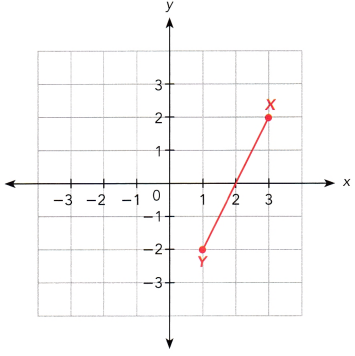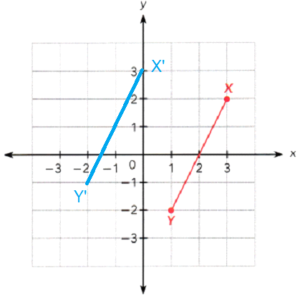Question 14.
Square ABCD is translated 2 units to the right and 2 units down.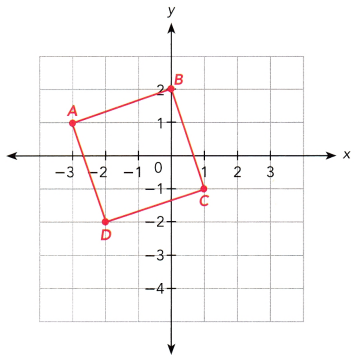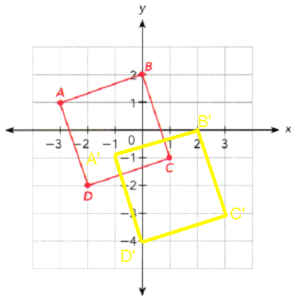Question 15.
On a coordinate plane, the coordinates of two points are A (3, 4) and B (3, 0). Point A’ is the image of point A and point 8′ is the image of point 8 under a reflection in the y-axis. (Lesson 8.2)

a) Find the coordinates of A’and B’.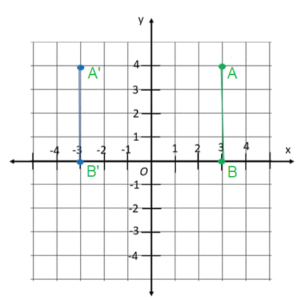b) Draw the image of the line segment OA, where O is the origin, under a reflection in the x-axis. Use 1 grid square on both axes to represent 1 unit for the interval from -4 to 4.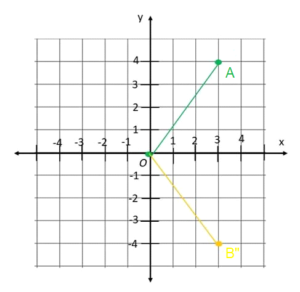Question 16.
Triangle ABC and trapezoid STUV are shown on the coordinate plane. On a copy of the graph, draw the images of triangle ABC under a reflection in the y-axis. Then draw the image of trapezoid STUV under a reflection in the x-axis. (Lesson 8.2)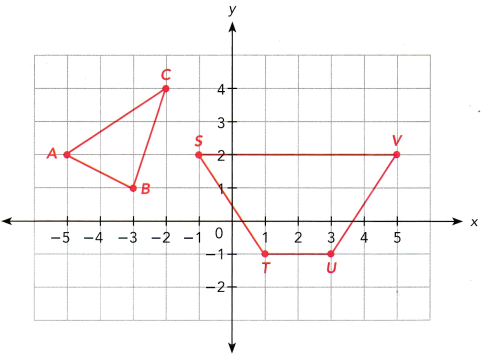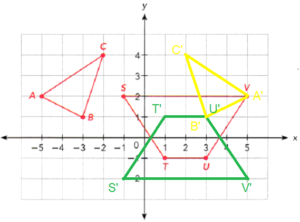Question 17.
A rotation of point A about the origin maps the point onto A’. State the angle and direction of rotation. (Lesson 8.3)

a)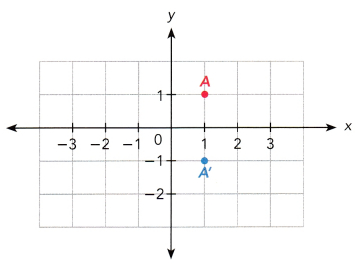Answer: The point A is on (1,1). The rotation of 90 degree clockwise, the coordinate should have changed from (x,y) to (y, -x). Since A’ is on (1, -1) from the original point (1,1) then it follows the rule for the 90 degree rotation in the clockwise direction.

b)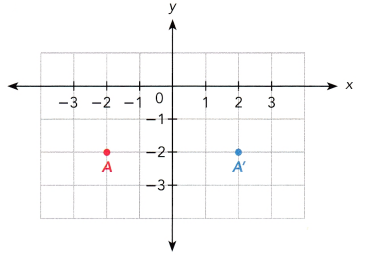Answer: Point A is on (-2,-2). The rotation of 90 degree counterclockwise, the coordinate shifts from (x,y) to (-y,x). Since A’ is on (2,-2) from the original point (-2,-2) then it follows the rule for the 90 degree rotation in the counterclockwise direction.

Question 18.
Triangle ABC has vertices A (2, 3), 8 (3, 1) and C (4, 2). Draw the triangle ABC and its image under a rotation of 180° about the origin. Use 1 grid square on both axes to represent 1 unit for the interval from -4 to 4. (Lesson 8.3)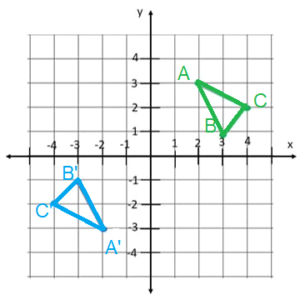Tell whether each transformation is a dilation. Explain. (Lesson 8.4)

Question 19.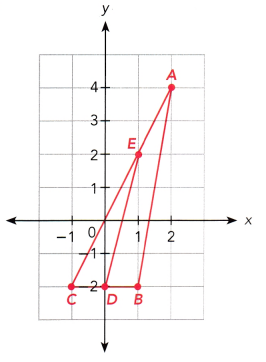Answer: The center of dilation is point C(or C’) since it is the point that did not change evn after the transformation. Also note that a transformation in dilation has a scale factor. The triangle A’B’C’ is 1/3 size compared to the original triangle, so the scale factor is 1/3. Therefore, the transformation is dilation.

Question 20.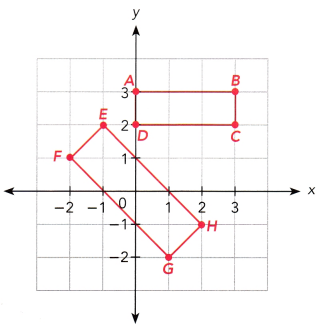Answer: Creating lines to connect points of the original rectangle to their corresponding points in their image to pinpoint the intersection of all points, the line won’t ever intersect. Thus, the center of dilation does not exist. Therefore, the transformation is not a dilation.

Question 21.
The figure described is mapped onto its image by a dilation with its center at the origin, O. Draw each figure and its image on a coordinate plane. (Lesson 8.4)

a) The vertices of triangle DEF are D (-3, -1), E (-3, -4), and F (-1, -3). Triangle DEF is mapped onto triangle D’E’F’ with scale factor -2.
Coordinates of D x scale factor
(-3,-1) x -2
(6,2)
Thus the coordinate of D’ is (6,2). Next E’ corresponds to E, then use the coordinates of E to determine the coordinates E’ after the dilation.
Coordinates of E x scale factor
(-3,-4) x -2
(6,8)
Thus, the coordinate of E’ is (6,8). Lastly, F’ corresponds to F so use the coordinates of F to determine the coordinates of F’ after the dilation.
Coordinates of F x scale factor
(-1,-3) x -2
(2,6)
Thus the F’ coordinate (6,6)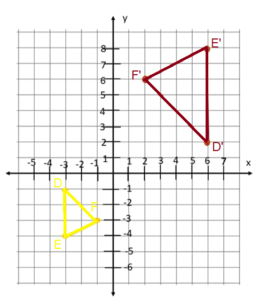b) The vertices of trapezoid ABCD are A (-2, -2), B (-2, -4), C (4, -4) and D (2, -2). Trapezoid ABCD is mapped onto trapezoid A’B’C’D’ with scale factor $$\frac{1}{2}$$.
Coordinate of A x scale factor
(-2,-2) x 1/2
= (-1,-1)
The coordinate of A’ is (-1,-1)
Coordinates of B x scale factor
(-2,-4) x 1/2
= (-1,-2)
The coordinate of B’ is (-1,-2)
Coordinates of C x scale factor
(4,-4) x 1/2
= (2,-2)
The coordinate of C’ is (2,-2)
Coordinates of D x scale factor
(2,-2) x 1/2
= (1,-1)
The coordinate of D is (1,-1)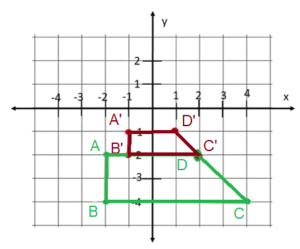Question 22.
The side lengths of a triangle are 3 inches, 6 inches, and 8 inches. The triangle undergoes a dilation. Find the side lengths of the ¡mage of the triangle for each of the scale factors in a) to d). Tell whether each dilation is an enlargement or a reduction of the original triangle. (Lesson 8.4)
a) 2
b) $$\frac{1}{4}$$
c) 120%
d) 1.6

a) Side length of 8 inches x scale factor
8 x 2
16
Side length of 6 inches x scale factor
6 x 2
12
Side length of 3 inches x scale factor
3 x 2
6
Therefore, the 3 inches side of the triangle grows into a 6 inches side.

b) At a scale of 1/4
Side length of 8 inches x scale factor
8 x 1/4
2
Side length of 6 inches x scale factor
6 x 1/4
1.5
Side length of 3 inches x scale factor
3 x 1/4
0.75
Therefore, the 3 inches side of the triangle shrinks into a 0.75 inch side.

c) The scale factor is in a percentage form, divide the give percent into 100 to convert it into decimal.
120/100 = 1.2
Thus, the scale factor is 1.3. So, at a scale of 1.2
Side length of 8 inches x scale factor
8 x 1.2
9.6
Therefore, the 8 inches side of the triangle grows into a 9.6 inches side.
Side length of 6 inches x scale factor
6 x 1/2
7.2
Therefore, the 6 inches side of the triangle grows into a 7.2 inches side.
3 x 1.2
3.6
Therefore, the 3 inches side of the triangle grows into a 3.6 inches side.

d) Scale factor is 1.6
Side length of 8 inches x scale factor
8 x 1.6
12.8
Therefore, the 8 inches side of the triangle grows into 12.8 side.
Side length of 6 inches x scale factor
6 x 1.6
9.6
Therefore, the 6 inches side of the triangle grows into 9.6 inches side
Side length of 3 inches x scale factor
3 x 1.6
4.8
Therefore, the 3 inches side of the triangle grows into a 4.8 inches side.

Question 23.
Triangle A with vertices (-1, 3), (-1, 4) and (-3, 3) is mapped onto triangle B. Then triangle B is mapped onto triangle C as shown on the coordinate plane. (Lesson 8.5)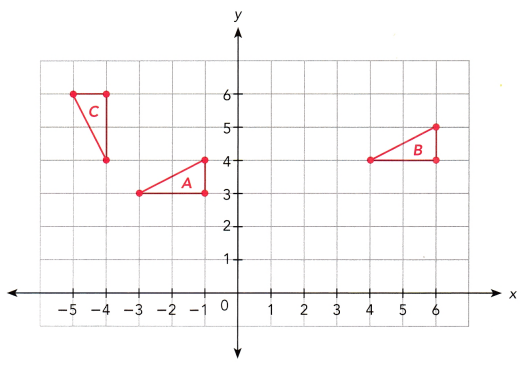a) Describe the transformation that maps triangle A onto triangle B.
Answer: The point (-3,3) of triangle A is mapped onto (4,4) by moving 1 unit up and 7 units to the right. For the points of triangle A, it also ascends once and moves 7 times to the right. This transformation that moves the original figure up or down or left or right is translation.

b) Describe the transformation that maps triangle B onto triangle C.
Answer: Points (4,4), (6,4) and (6,5) mapped onto (-4,4),(-4,6) and (-5,6) changes the coordinates from its original for (x,y) into (-y,x). The transformation that changes the form of the coordinates from (x,y) into (-y,x) is a 90-degree rotation at the origin in a counterclockwise direction.

Question 24.
State whether the triangles are congruent. If they are congruent, write the statement of congruence and state the test used. (Lesson 9.1)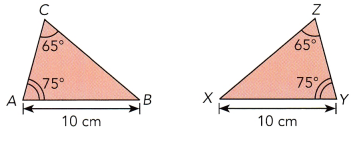Answer: AB corresponds to XY and both the measures 10 cm, Triangle A corresponds to Triangle Y while Triangle C corresponds to Triangle Z and this two corresponding angles are congruent by Angle Angle Side Test which states that if the two corresponding angles of two triangles are congruent and any of their side are also congruent, then the triangles are congruent

Question 25.
∆AMS is congruent to ∆ERN. Find the values of x and y. (Lesson 9.1)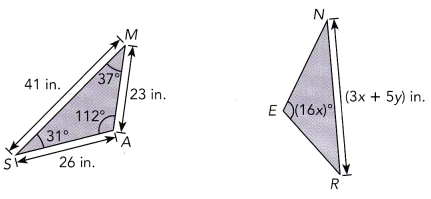To angle A of Triangle AMS and angle E of Triangle ERN are equal.
Angle A = Angle E
The measure of angle A is equals to 112 degree while measure of angle E is 16x degree
112 = 16x
112/16 = x
7 = x
Therefore, x is equal to 7
SM of triangle ASM and NR of triangle ERN are equal.
SM = NR
Measure of SM equals to 41 inches while measure of NR is (3x+5y)inches.
41 = (3x+5y)
using x = 7,
41 = 3(7) + 5y
41 = 21 + 5y
5y = 41 – 21
5y = 20
y = 20/5
y = 4

Triangle ABC is similar to triangle POR. Find the value of a. (Lesson 9.2)

Question 26.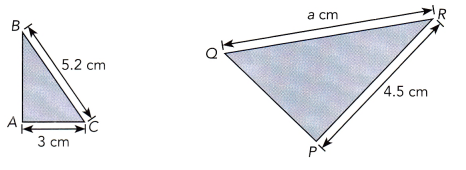AC measuring 3 cm corresponds to PQ whose measure is 4.5 cm and CB measuring 5.2 corresponds to QR which measure a. Since the triangles are similar then their corresponding sides are proportional thus,
AC/PQ = CB/QR
3/4.5 = 5.2/a
3a = 23.4
a = 23.4/3
a = 7.8
Therefore, a is equal to 7.8

Name the test you can use to determine whether the two triangles are similar. Then find the value of x. (Lesson 9.2)

Question 27.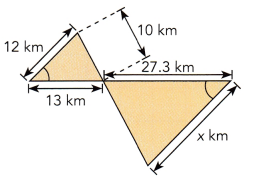Triangle ROS and POQ are vertical angles, and pair of opposite angles are formed due to an intersection of line is equal. Observe that angle R is congruent to angle P. Third corresponding angle is also equal. As there are two pairs of corresponding angles in Triangle ROS and POQ, third corresponding angle is also equal. By the Angle-Angle-Angle similarity theorem, which states ate if all the corresponding angles of two triangles are similar, that two triangles are similar.
Similarity of the triangles are proven, to solve x, RS which measures 12 km corresponds to PQ measuring x km and RO whose length is 13 km corresponds to PO measuring 27.3 km
RS/PQ = RO/PO
12/x = 13/27.3
12 x 27.3 = 13x
327.6 = 13x
x = 327.6/13
x = 25.2
Therefore, x = 25.2 km

Solve on a coordinate grid. (Lesson 9.3)

Question 28.
∆ABC undergoes two transformations to form the image ∆A”B”C”.
a) What are the coordinates of ∆A”B”C” if ∆ABC is first translated 2 units to the right and 3 units down, and then reflected in the line x = 2?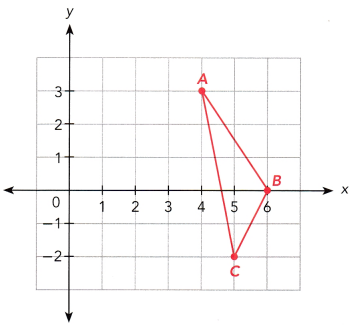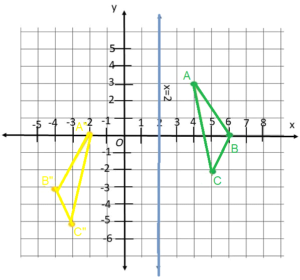b) What are the coordinates of ∆A”B”C” if ∆ABC is first reflected in the line x = 2, and then translated 2 units to the right and 3 units down?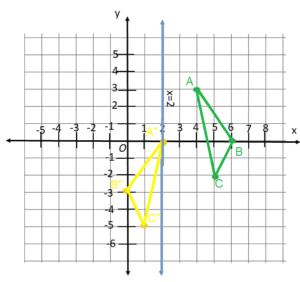c) Do the two triangles ∆A”B”C” described in a) and b) have the same coordinates? Are they congruent? Explain.
The two traingles are congruent since the only transformation is a reflection and transformation which is known for flipping the original images while translation is known for moving image up and down and left to right. So the size of triangle is still the same and are just plotted at different locations. For their coordinate, the locations are transformed twice, both depends on the first trnasformations that the triangles undergo. Triangle A”B”C” are on Quadrant III while the second one is on Quadrant IV. Therefore, their coordinates are not congruent.

Problem Solving

Question 29.
A pool table has a width of 5.5 inches. A ball travels a distance of 12.3 inches diagonally from one corner to the other corner of the table. Find the length of the pool table, rounded to the nearest tenth. (Chapter 7)
Using pythagorean theorem,
(5.5)² + j² = (12.3)²
30.25 + j² = 151.29
j² = 151.29 – 30.25
j² = 121.04
j ~ 11 (Square root of 121.04)
Therefore, the length of the pool table is 11 inches.

Question 30.
When a drawbridge is open, the bridge forms a right angle with the doorway it leads to. A straight chain connects the far end of the bridge to the top of the doorway. If the doorway is 2 meters high and the chain is 2.5 meters long, find the length of the bridge, rounded to the nearest tenth. (Chapter 7)
Using the pythagorean theorem,
y² + z² = x²
(2.5)² + 2² = x²
6.25 + 4 = x²
10.25 = x²
x ~ 3.2 (square root of 10.25)
Therefore, the drawbridge is around 3.2 m in length

Question 31.
Gabriel photocopied an exact copy of the shape ABCDE. The photocopied shape is shown on the right. (Chapter 9)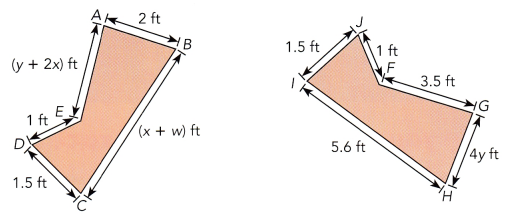a) Name the shape congruent to ABCDE.
Answer: Point A corresponds to G , B point to H and point C corresponds to I, D corresponds to J and E corresponds to F. Therefore, the congruent shape to ABCDE is GHIJF.

b) Find the values of w, x, and y.
Since ABCDE is congruent to GHIJF, their corresponding angles are equal. Thus, side AB of ABCDE is equal to GH of GHIJF, since these two sides corresponds to each other. So,
AB = GH
The measure of the lengths of AB is 2 feet while GH is 4y feet.
2 = 4y
y = 2/4
y = 0.5
Therefore, y is equal to 0.5
Side EA of ABCDE is equal to FG of GHIJF
EA = FG
The measure of the lengths of EA is (y+2x) feet while FG is 3.5 feet
y + 2x = 3.5
0.5 + 2x = 3.5
2x = 3.5 – 0.5
2x = 3
x = 3/2
x = 1.5
Therefore, x is equal to 1.5
Side BC of ABCDE is equal to HI of GHIJF
BC = HI
The measure of lengths of BC is (x + w) feet while HI is 5.6 feet
x + w = 5.6
1.5 + w = 5.6 (As x = 1.5)
w = 5.6 – 1.5
w = 4.1
Therefore, w is equal to 4.1

Solve. Show your work. (Chapters 7, 9)

Question 32.
Mary wants to pour 130 cm3 of water into the conical cup shown.

a) Find the volume of the conical cup. Use 3.14 as an approximation for π. Round your answer to the nearest tenth.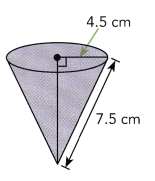Using pythagorean theorem,
(4.5)² + m² = (7.5)²
20.25 + m² = 56.25
m² = 56.25 – 20.25
m² = 36
m = 6
Therefore, the height of the cone is 6 cm
For the volume of the cone, 3.14 is the value of π and m is the height,
Volume of the cone = 1/3 Area of the base x Height of the cone
= 1/3πr² x m
= 1/3 x (3.14) x (4.5)² x (6)
= 127.17
Therefore, cone has a volume of 127.17 cubic cm.

b) Will the water overflow? Explain.
Answer: Since Mary wants to pour 127.17 cubic centimeters, the water will spill. The conical cup can only accommodate up to 127.17 cubic centimeters of liquid.

Question 33.
Two cones are similar in shape. The ratio of the diameters of their bases is 2 : 7. The radius of the smaller cone is 4.5 inches.

a) Find the radius of the larger cone.
Convert the ratio 2:7 into fractions, then the result will be 2/7. Let s be the unknown measure of the radius, so
2/7 = 4.5/s
2 x s = 4.5 x 7
2s = 31.5
s = 31.5/2
s = 15.75
Therefore, the larger cone has a radius measuring 15.75 inches.

b) Write the ratio of the height of the smaller cone to the height of the larger cone.
Answer: Height of the smaller cone / Height of the larger cone

Solve. Show your work. (Chapters 8, 9)

Question 34.
A car is 25 feet long and 6 feet wide. A model of the same car is 9 inches long. What is the width of the model of the car if the model is a dilation of the car?
Scale factor = Length of the model car/ Length of the actual car
= 0.75/25
= 0.03
Thus, the scale factor is 0.03
To solve width, multiplying the actual width to the scale factor,
Width of the model car = Width of the car x scale factor
= 6 x 0.03
= 0.18
Therefore, the width of the model car is 0.18 feet,
Converting this into inches, multiplying 0.18 with 12
Thus, the model car has a width of 2.16 inches.

Question 35.
Figure PQRST is dilated with center P and a scale factor of 1.45. Under this dilation, it is mapped onto figure UVWXY. Figure UVWXYis then mapped onto ABCDE by a reflection about segment UV.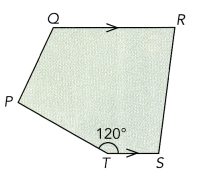a) Tell whether PQRST and ABCDE are congruent or similar figures. Explain.
Answer: The reflection that maps UVWXYZ onto ABCDE does not change the size and shape of the figure. Thus, they are congruent. The dilation that maps PQRST onto UVWXYZ however enlarges the size of PQRST. Therefore, UVWXYZ and PQRST are similar.

b) Find m∠UYX.
Answer: UVWXYZ and PQRST are congruent, then their corresponding angles are equal. The angle P corresponds to angle U, Q to angle V and angle R corresponds to W. The corresponding angle of S is angle X and angle T corresponds to Y . Since angle T corresponds to angle Y, then T is equal to Y. Angle T is equal to 120 degree. Therefore, angle Y is equal to 120 degree.

c) The area of PQRST is 20 square inches. Find the area of ABCDE.
Answer: The area of ABCDE is also a dilated image of PQRST. So, by multiplying the area of the actual image which is 20 square inches to the scale factor, the area of ABCDE
Area of ABCED = Area of PQRST x Scale factor
= 20 x 1.45
= 29
Therefore, ABCDE has an area of 29 square inches.